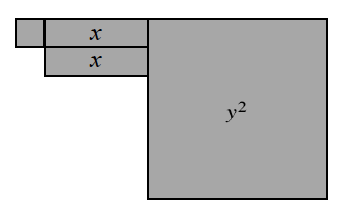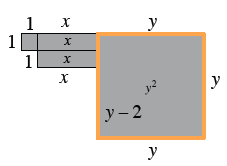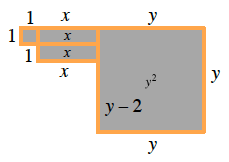### Home > AC > Chapter 2 > Lesson 2.1.7 > Problem2-71

2-71.Examine the shape made with algebra tiles at right.

1. Write an expression that represents the perimeter of the shape. Then evaluate your expression for $x=6$ and $y=10$ units.

Label each side with its length and write an expression for the perimeter. $2x+3y+(y−2)+4=2x+4y+2$Evaluate this expression.
$2(6)+4(10)+2\\12+40+2=54$

$54$

2. Write an expression that represents the area of the shape. What is the area if $x=6$ and $y=10$ units?

Area is the total square units of a shape.

It might be helpful to break the shape into parts, find the area of the parts, and then sum them to find the area of the whole.$113$ square units. Make sure to show your work, including the expression you wrote for the area.